Courses

# Magnetic Circuits of Electrical Machines

## 10 Questions MCQ Test Topicwise Question Bank for Electrical Engineering | Magnetic Circuits of Electrical Machines

Description
This mock test of Magnetic Circuits of Electrical Machines for Electrical Engineering (EE) helps you for every Electrical Engineering (EE) entrance exam. This contains 10 Multiple Choice Questions for Electrical Engineering (EE) Magnetic Circuits of Electrical Machines (mcq) to study with solutions a complete question bank. The solved questions answers in this Magnetic Circuits of Electrical Machines quiz give you a good mix of easy questions and tough questions. Electrical Engineering (EE) students definitely take this Magnetic Circuits of Electrical Machines exercise for a better result in the exam. You can find other Magnetic Circuits of Electrical Machines extra questions, long questions & short questions for Electrical Engineering (EE) on EduRev as well by searching above.
QUESTION: 1

### What is the inductance of a coil in which a current of 0.1A increasing at the rate of 0.5 A/s represents a power flow of 0.5 watt?

Solution:

Power flow through the coil is: p = ei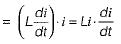or,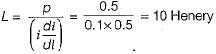QUESTION: 2

### A coil of 100 turns is wound on a torroidal magnetic core having a reluctance of 104 AT/Wb. When the coil current is 5 A and is increasing at the rate of 200 A/s, the voltage across the coil would be (assume coil resistance to be 2 Ω)

Solution: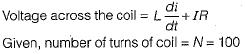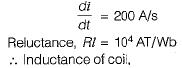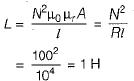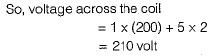QUESTION: 3

### The field winding of a dc electro-magnet is wound with 960 turns and has a resistance of 50 Ω. The excitation voltage is 230 V and the magnetic flux linking the coil is 5 mWb. The energy stored in the magnetic field is

Solution:

Current through the field winding,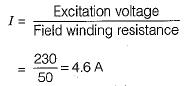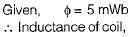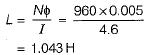So, energy stored in magnetic field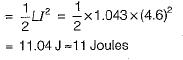QUESTION: 4

The flux in a magnetic core is alternating sinusoidally at a frequency of 600 Hz. The maximum flux density is 2 Tesla and the eddy current loss is 15 Watts. What would be the eddy current loss in the core if the frequency is raised to 800 Hz and the maximum flux density is reduced to 1.5 Tesla?

Solution:

We know that eddy current loss,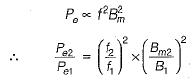or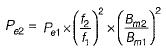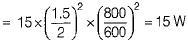QUESTION: 5

Two inductances of 15 mH and 25 mH are connected in series such that their fluxes oppose each other. They are so placed that the coefficient of coupling is 0.8. The value of mutual inductance between them will be

Solution: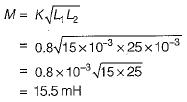QUESTION: 6

The property of a material which opposes the production of magnetic flux in it is known as:

Solution:

Resistance in electrical circuit corresponds to reluctance in magnetic circuit. Reluctance opposes the production of magnetic flux in a magnetic circuit and resistance opposes the flow of current in electrical circuit.

QUESTION: 7

Air exhibits

Solution:
QUESTION: 8

The laws of electromagnetic induction are summarized in the following equation:

Solution:

From Faraday’s law of electromagnetic induction,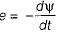(minus sign is due to Lenz’s law).

QUESTION: 9

EMF induced in a coil rotating in a uniform magnetic field will be maximum when the

Solution: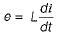Hence, emf induced is maximum when di/dt is maximum.

QUESTION: 10

The emf induced in a conductor rotating in a bipolar field is

Solution:

As the field is bipolar, therefore the induced emf will be alternating in nature.